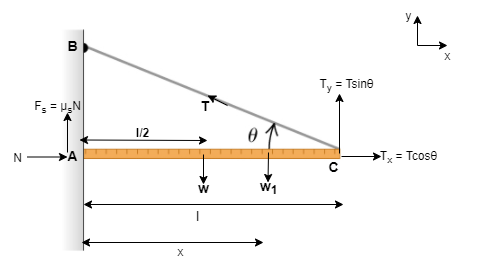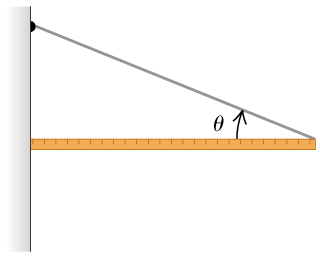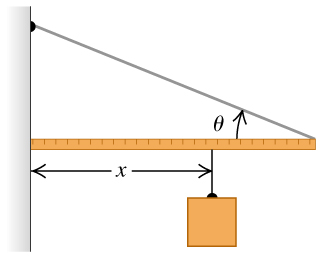# Problem: One end of a uniform meter stick is placed against a vertical wall.The other end is held by a lightweight cord that makes an angle θheta with the stick. The coefficient of static friction between the end of the meter stick and the wall is 0.38.Part A. What is the maximum value the angle θheta can have if the stick is to remain in equilibrium?Part B. Let the angle between the cord and the stick is θexttip{heta }{theta} = 15°. A block of the same weight as the meter stick is suspended from the stick, as shown, at a distance x from the wall. What is the minimum value of x for which the stick will remain in equilibrium?Part C. When θexttip{heta }{theta} = 15°, how large must the coefficient of static friction be so that the block can be attached 13 cm from the left end of the stick without causing it to slip?

###### FREE Expert Solution

We can visualize our problem with the block added in the diagram below:Where:

μs is the coefficient of static friction.

N is the normal force from the wall on the meterstick.

Fs is the static friction from the wall on the meterstick.

w is the weight of the meter stick at the center of gravity.

w1 is the weight of the block.

T is the tension from the cord on the meter stick.

l is the length of the meter stick.

x is the distance from the wall to the position of the block on the meter stick.

Part A.

When no mass is hung on the meter stick, w1 = 0

The equilibrium condition is given by:

$\overline{)\begin{array}{rcl}\mathbf{\sum }\mathbf{F}& {\mathbf{=}}& {\mathbf{0}}\\ \mathbf{\sum }\mathbf{\tau }& {\mathbf{=}}& {\mathbf{0}}\end{array}}$

Torque:

, $\overline{){\mathbit{\tau }}{\mathbf{=}}{\mathbit{r}}{\mathbit{F}}}$

Let's consider the net torque at point C,###### Problem Details

One end of a uniform meter stick is placed against a vertical wall.The other end is held by a lightweight cord that makes an angle θ with the stick. The coefficient of static friction between the end of the meter stick and the wall is 0.38.

Part A. What is the maximum value the angle θ can have if the stick is to remain in equilibrium?

Part B. Let the angle between the cord and the stick is θ = 15°. A block of the same weight as the meter stick is suspended from the stick, as shown,at a distance x from the wall. What is the minimum value of x for which the stick will remain in equilibrium?

Part C. When θ = 15°, how large must the coefficient of static friction be so that the block can be attached 13 cm from the left end of the stick without causing it to slip?